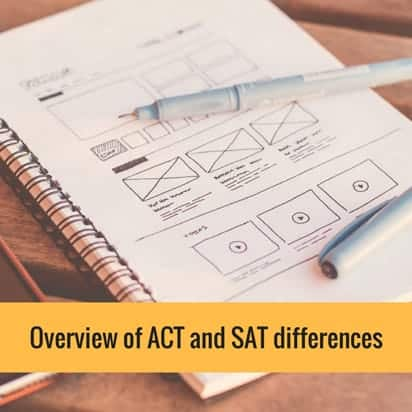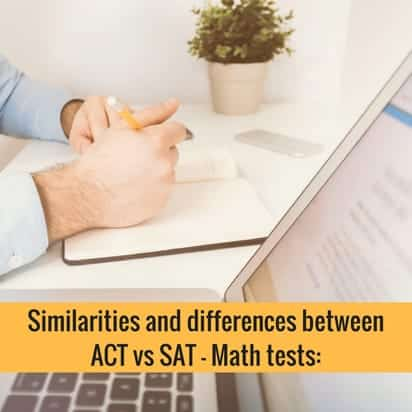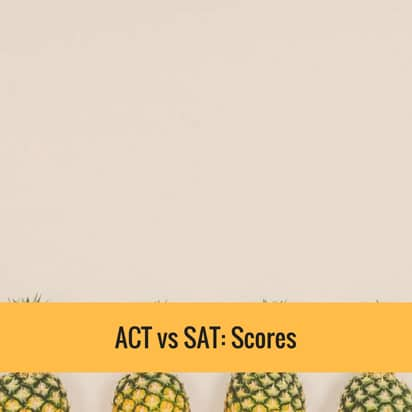# ACT vs SAT: A Comparison On Basic Structural And Logistical Differences & ACT vs SAT: Scores

The ACT and SAT tests generally contain similar types of content. Both are used for college admissions and applying for scholarships. The huge difference between the ACT vs SAT test is that the ACT has a science test while SAT doesn’t have this. Also, the SAT Math section doesn’t allow calculator use, unlike the ACT. Nonetheless, colleges in the U.S. don’t prefer one over the other, so you must explore both ACT and SAT tests to determine which one is ideal for you.

## How to Choose ACT vs SATThe best way to determine if the ACT or SAT is ideal for you is to take a full-length practice test in each of the tests. Considering the content and style of the ACT and SAT tests are similar, factors like what types of questions you find most challenging as well as how you handle time pressure can help you decide which test is most suitable.

For instance, the SAT test’s pace gives you a bit more time to analyze the questions, while taking an ACT test will put you under pressure. A number of students prefer the extra time to ponder a question, while others really do well under the time pressure.

Despite all the similarities of ACT and SAT tests, there are still a number of ways in which the tests differ from each other. The ACT test is overall slightly shorter compared to the SAT test. Also, the time limits and the number of questions are contrasting for corresponding sections.

Check our ACT Practice Tests for English

## Here is an overview of the basic structural and logistical differences between ACT vs SAT:SAT ACT Reason To Take The Test Students use test scores for college admissions as well as merit-based scholarships. Students use test scores for college admissions as well as merit-based scholarships. Structure Of The Test Math Writing and Language Reading Essay (Optional) Math Reading Science Reasoning English Essay (Optional) Duration 3 hours and 50 minutes (with essay) 3 hours (without essay) 3 hours and 40 minutes (with essay) 2 hours and 55 minutes (without essay) Essays – ACT vs SAT The essay will test student’s comprehension of a source text. The essay will test how well a student analyzes and evaluate complex problems. Reading Passages 5 4 Math Algebra I & II Arithmetic Geometry, Trigonometry, and Data Analysis Algebra I & II Arithmetic Geometry and Trigonometry Calculator Policy Students are allowed to use a calculator on some math questions. Students can utilize calculator on all math questions. Science Section None 1 science section to test the student’s critical thinking skills

Related Topic: SAT Practice Tests

 Order of Test Sections 1. Reading 2. Writing and Language 3. Math No Calculator 4. Math Calculator 5. Essay (optional) 1. English 2. Math 3. Reading 4. Science 5. Writing (optional) Time Per Test Section Reading: 65 mins Writing and Language: 35 mins Math (without calculator): 25 mins Math (with calculator): 55 mins Essay (optional): 50 mins English: 45 mins Math: 60 mins Reading: 35 mins Science: 35 mins Writing (optional): 40 mins Number of Test Questions Reading: 52 questions Math with Calculator: 38 questions Math without Calculator: 20 questions Writing and Language: 44 questions Essay (optional): 1 essay Reading: 40 questions English: 75 questions Science: 40 questions Math: 60 questions Writing (optional): 1 essay How Much Will You Pay? \$60 with Essay \$46 without Essay \$58.50 with Writing \$42.50 without Writing Who Accepts ACT and SAT Test Scores? All United States Colleges and Universities All United States Colleges and Universities

## Additional information about the similarities and differences between ACT vs SAT – Math tests:• The new SAT test has leveled up its game. Students will encounter some questions on advanced Math as well Trigonometry. Nonetheless, as SAT expert Chris Lele reported that at least right now, students who will take the ACT Math test will see more questions in the realm of Trigonometry and Algebra II. All of the Trigonometry tests on either the ACT or SAT is at a very basic level.
• Usage of Calculator: Test-takers can utilize a calculator on every single question on the ACT Math test. However, the SAT Math test will be about 25 minutes with 20 questions and no calculator. The SAT Math test is easy enough for test-takers, but it is noteworthy that test-takers might want to refresh their mental Math skills. Nonetheless, if you are extremely clever with numbers and can do a mathematical calculation in your head, you might stand out on the SAT Math test over many of your peers.
• Multiple Choice vs  No answer choices: All of the ACT Math tests contain multiple choice questions, which means test-takers will have at least a 20% of chance of getting the correct answer – even if the students have no idea what they are doing. Meanwhile, the SAT Math test has 20% grid-ins and 80% multiple choice, which means test-takers will have to fill in the blanks with their own answers.

Related Topic: SAT Math Practice Test

### ACT vs SAT: Scores• The ACT uses a composite score to provide test-takers an overall score. The overall composite score would range from 1 to 36, and it would be an average of the scores on each of the multiple-choice sections. Students will also receive their individual section scores, but for most colleges in the United States, it is the student’s composite score that matters.

So, for instance, if you received a 32 on Math, 28 on Reading, 24 on Science and 25 on English, your overall composite score would be (32 + 28 + 24 + 25)/4 = 27.5 – this will be rounded to the nearest whole number – 28.

• The SAT score ranges between 400 and 1600, which is based on adding the student’s Math score from 200-800 and Reading/Writing score from 200-800 together.

It is noteworthy that even though the SAT test has three main multiple-choice sections (Reading, Math, and Writing), SAT will combine Writing and Reading into one score out of 800. This is much different from the old SAT test, on which test-takers will receive a score out of 800 on each of the three main multiple-choice sections, which means students will receive the highest score of 2400 on the old SAT test.

See our post here if you want to know the difference between Old SAT vs New SAT.

Related Topics:

Watch our Online GED Math Videos covering all topics you will face during the GED Math test